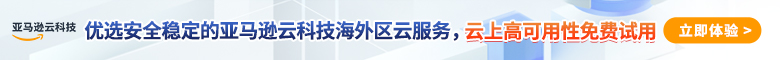# 17个常用的正则表达式规则（涵盖大部分常规需要的）1、匹配中文字符

``````[\u4e00-\u9fa5]
``````

2、匹配双字节字符

``````[^\x00-\xff]
``````

3、匹配空白行

``````\n\s*\r
``````

4、匹配Email地址

``````[\w!#\$%&'*+/=?^_`{|}~-]+(?:\.[\w!#\$%&'*+/=?^_`{|}~-]+)*@(?:[\w](?:[\w-]*[\w])?\.)+[\w](?:[\w-]*[\w])?
``````

5、匹配URL

``````[a-zA-z]+://[^\s]*
``````

6、匹配国内电话号码

``````\d{3}-\d{8}|\d{4}-\{7,8}
``````

7、匹配QQ号码

``````[1-9][0-9]{4,}
``````

8、匹配中国邮政编码

``````[1-9]\d{5}(?!\d)
``````

9、匹配18位身份证号码

``````^(\d{6})(\d{4})(\d{2})(\d{2})(\d{3})([0-9]|X)\$
``````

10、匹配（年-月-日）日期格式

``````([0-9]{3}[1-9]|[0-9]{2}[1-9][0-9]{1}|[0-9]{1}[1-9][0-9]{2}|[1-9][0-9]{3})-(((0|1)-(0[1-9]|[0-9]|3))|((0|11)-(0[1-9]|[0-9]|30))|(02-(0[1-9]|[0-9]|2[0-8])))
``````

11、匹配正整数

``````^[1-9]\d*\$
``````

12、匹配负整数

``````^-[1-9]\d*\$
``````

13、匹配整数

``````^-?[1-9]\d*\$
``````

14、匹配非负整数

``````^[1-9]\d*|0\$
``````

15、匹配非正整数

``````^-[1-9]\d*|0\$
``````

16、匹配浮点数

``````^[1-9]\d*\.\d*|0\.\d*[1-9]\d*\$
``````

17、匹配负浮点数

``````^-[1-9]\d*\.\d*|-0\.\d*[1-9]\d*\$
``````